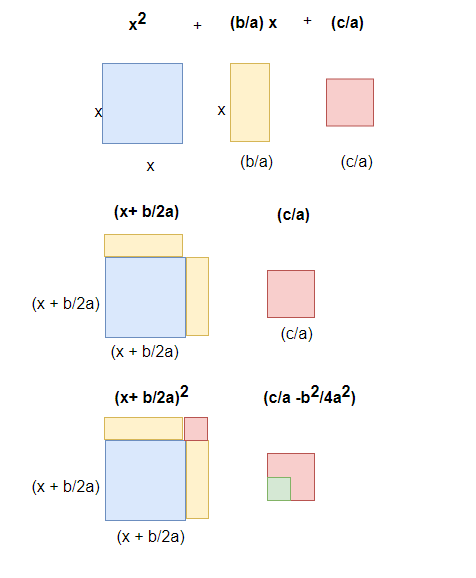# How to solve quadratic equations by completing the square

## Solving quadratic equations by completing the square

In this method we create square on LHS and RHS and then find the value.
ax2 +bx+c=0
Step 1 Dividing by a on both the sides
$x^2 +\frac {b}{a} x+\frac {c}{a}=0$

Step 2 Square formation on the LHS using the first two terms. So quadratic equations becomes
$(x+ \frac {b}{2a})^2 - (\frac {b}{2a})^2 + \frac {c}{a}=0$

Step 3 Rearranging the terms on the LHS and RHS,we get the quadratic in the form
$(x+ \frac {b}{2a})^2=\frac {(b^2-4ac)}{4a^2}$

Step 4 Now you can see that RHS does not contain any variable ,it just contains the variables,So we find the square root of the calculated term and RHS to find the roots of the quadratic equation

## Geometric Meaning of completing the square

This is explained Geometrically as below## Completing square method ExampleExample 1 $x^2 +4x-5=0$Solutionstep 1 Now a=1,so no need to dividestep 2 Square formation on the LHS using the first two terms. So quadratic equations becomes $(x+2)^2 -4-5=0$step 3 Rearranging the terms on the LHS and RHS,we get the quadratic in the form $(x+2)^2=9$step4 Roots of the equation can be find using square root on both the sides $x+2 =-3$ => $x=-5$ $x+2=3$ => $x=1$Example 2 $x^2-9x+20=0$Solutionstep 1 Now a=1,so no need to dividestep 2 Square formation on the LHS using the first two terms. So quadratic equations becomes $(x-4.5)^2 +20 -20.25=0$step 3 Rearranging the terms on the LHS and RHS,we get the quadratic in the form $(x-4.5)^2=.25$step4 Roots of the equation can be find using square root on both the sides $x-4.5 =.5$ => $x=5$ $x - 4.5=-.5$ => $x=4$Example 3 $x^{2} + 4 x - 45 = 0$Solutionstep 1 Now a=1,so no need to dividestep 2 Square formation on the LHS using the first two terms. So quadratic equations becomes $(x+2)^2 -45-4=0$step 3 Rearranging the terms on the LHS and RHS,we get the quadratic in the form $(x+2)^2=49$step4 Roots of the equation can be find using square root on both the sides $x+2 =-7$ => $x=-9$ $x+2=7$ => $x=5$Solve below questions using Completing square method $x^{2} - 12 x + 35 = 0$ $x^{2} - 14 x + 40 = 0$ $y^{2} - 4 y + 4 = 0$ $z^{2} - 6 z + 8 = 0$ Answer $x = -9, x = 5$ $x = 5, x = 7$ $x = 4, x = 10$ $x = 2$ $x = 2, x = 4$

Check out the below Video to see more examples on Square MethodGo back to Class 10 Main Page using below links

### Practice Question

Question 1 What is $1 - \sqrt {3}$ ?
A) Non terminating repeating
B) Non terminating non repeating
C) Terminating
D) None of the above
Question 2 The volume of the largest right circular cone that can be cut out from a cube of edge 4.2 cm is?
A) 19.4 cm3
B) 12 cm3
C) 78.6 cm3
D) 58.2 cm3
Question 3 The sum of the first three terms of an AP is 33. If the product of the first and the third term exceeds the second term by 29, the AP is ?
A) 2 ,21,11
B) 1,10,19
C) -1 ,8,17
D) 2 ,11,20